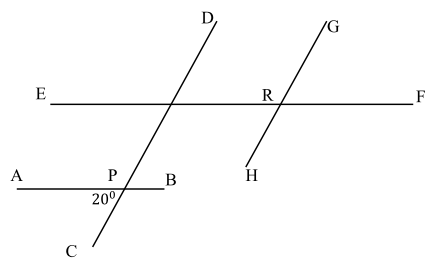# Free SAT Test Questions

Question 1 of 1
ID: SAT-AM-2
Section: Math - Additional Topics in Math
Topic: Geometry
Difficulty level: Easy

(Practice Mode: Single selected Question » Back to Overview)

In the given figure below, $AB{\parallel}EF$, $CD{\parallel}GH$ and points P, Q and R are intersection points of the lines. If $\angle APC=20°$, what is the measurement of $\angle GRF$ (in degrees)? (Without calculator)